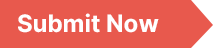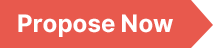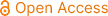### American Journal of Mathematical and Computer Modelling

Archive
• 2023, Volume 8

• 2022, Volume 7

• 2021, Volume 6

• 2020, Volume 5

• 2019, Volume 4

• 2018, Volume 3

• 2017, Volume 2

• 2016, Volume 1

Submit a Manuscript

Publishing with us to make your research visible to the widest possible audience.Propose a Special Issue

Building a community of authors and readers to discuss the latest research and develop new ideas.### A Comparative Analysis of Ordinary Least Squares and Quantile Regression Estimation Technique

This study explores quantile regression estimation technique and its practicality in regression analysis; hence we provide a comparative study in view of quantile regression as an alternative to the traditional ordinary least squares regression. Although the ordinary least squares (OLS) model examines the relationship between the independent variable and the conditional mean of the dependent variable, whereas the quantile regression model examines the relationship between the independent variable and the conditional quantiles of the dependent variable. Quantile regression overcomes various problems associated with OLS. First, quantile regression is defined and its advantages over ordinary least squares regression are illustrated. Also, specific comparisons are made between ordinary least squares and quantile regression estimation methods. Lastly, both estimation techniques were applied on a real life data and the results obtained from the analysis of two types of datasets in this study suggests that quantile regression provides a richer characterization of the data giving rise to the impact of a covariate on the entire distribution of the response variables as the effect can be very different for different quantiles. Quantile regression therefore gives an efficient and more complete view of the relationship amongst variables, hence, suitable in examining predictors effects at various locations of the outcome distribution.

Regression, Ordinary Least Squares Regression, Quantile Regression, Mean Square Error, Variance

Runyi Emmanuel Francis, Maureen Tobe Nwakuya. (2022). A Comparative Analysis of Ordinary Least Squares and Quantile Regression Estimation Technique. American Journal of Mathematical and Computer Modelling, 7(4), 49-54. https://doi.org/10.11648/j.ajmcm.20220704.11